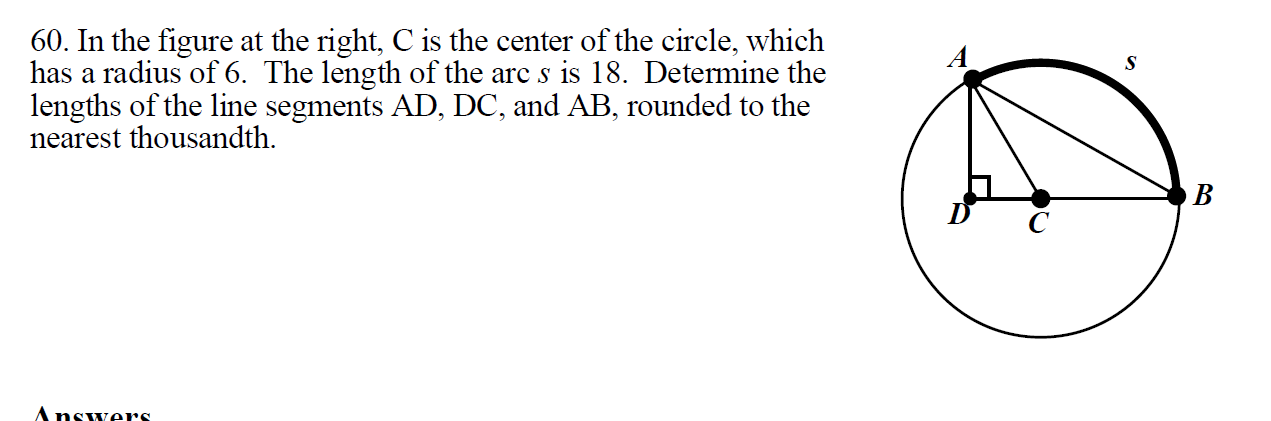# 60. In the figure at the right, C is the center of the circle, whichhas a radius of 6. The length of the arc s is 18. Detemine thelengths of the line segments AD, DC, and AB, rounded to thenearest thousandth.SBAnswers.

Questionhelp_outlineImage Transcriptionclose60. In the figure at the right, C is the center of the circle, which has a radius of 6. The length of the arc s is 18. Detemine the lengths of the line segments AD, DC, and AB, rounded to the nearest thousandth. S B Answers. fullscreen
check_circleExpert Solution
Step 1

Length of the arc, s = 18

Hence, angle corresponding to the arc AB = angle ACB = s / r = 18 / 6 = 3 radians = 3 x 180 / π = 172 degrees

Step 2

In the right angeld triangle, ADC

Angle ACD = ϴ = 180° - 172° = 8°

AC = r

Hence, AD = rsinϴ = 6 x ...

### Want to see the full answer?

See Solution

#### Want to see this answer and more?

Solutions are written by subject experts who are available 24/7. Questions are typically answered within 1 hour*

See Solution
*Response times may vary by subject and question
Tagged in

### Calculus